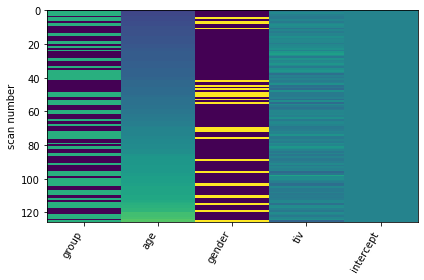# Nistats.reporting.make_glm_report computes different threshold than nistats.thresholding.map_threshold

For my second level analysis I use both `nistats.reporting.make_glm_report` to get a peak table and `nistats.thresholding.map_threshold` in order to obtain an unthresholded statistical image and a corresponding threshold. However, when I compare the computed threshold from `make_glm_report` and `map_threshold`, they are different. Both functions are provided with the same arguments for `alpha`, `height_control` and `cluster_threshold`. The only difference is, that `make_glm_report` is provided with `min_distance=8` (which shoulnd’t influence the computed threshold?) and that `map_threshold` is provided with a `mask_img`, where `mask_img ` is a grey-matter-mask (I am only interested in grey-matter-differences).

I am not 100% sure, why the thresholds are different, but I suspect that it might have something to do with the fact, that `mask_glm_report` does not include a `mask_img` argument, and so the analysis is run on the whole brain in contrast to `map_threshold` where the threshold is computed based on grey-matter-voxels only?

Here’s my code:

``````## Custom Settings ############################################################

SMOOTHING_FWHM = 8
ALPHA = 0.05
TWO_SIDED = False
HEIGHT_CONTROL = 'fdr'
CLUSTER_THRESHOLD = 0

## Create Design Matrix #######################################################

intercept = np.ones(sample_df.shape)
group = sample_df['group_boolean']
age = sample_df['age']
gender = sample_df['gender_boolean']
tiv = sample_df['tiv']
design_matrix = pd.DataFrame(np.vstack((group, age, gender, tiv, intercept)).T,
columns=['group','age','gender','tiv','intercept'])

ax = plot_design_matrix(design_matrix)
````````````## Conduct Second Level Analyses ##############################################

# Set up Model
smoothing_fwhm=SMOOTHING_FWHM,
)

second_level_model.fit(second_level_input=sample_df['filepath'].tolist(),
design_matrix=design_matrix)

z_img = second_level_model.compute_contrast(second_level_contrast='age',
second_level_stat_type='t',
output_type='z_score')

# get thresholded image and threshold (computed threshold ~ 4.3)
z_img_thresholded, threshold = map_threshold(stat_img=z_img,
alpha=ALPHA,
height_control=HEIGHT_CONTROL,
cluster_threshold=CLUSTER_THRESHOLD,
two_sided=TWO_SIDED)

# create report
report = make_glm_report(model=second_level_model,
contrasts='age',
alpha=ALPHA,
height_control=HEIGHT_CONTROL,
cluster_threshold=CLUSTER_THRESHOLD,
min_distance=8
)

report.open_in_browser()
``````

Threshold computed by `make_glm_report`:

Threshold (computed) = 2